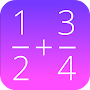# Fractions Math Pro APK

Fractions calculator: calculate, convert, simplify and compare fractions!.
50+
Thousand
4.3
457+
Rating
04/13/16
Last updated
1.6+
Android version
0.27 Mb
App size
Everyone
Content rating
\$0.99Free
Price
This calculator is the pro version of 'Fractions Math', and completely without advertisement.

This math app is a fractions calculator with various functions:

- add, subtract, multiply and divide fractions.
- convert fractions in decimals,
- simplify fractions
- compare fractions
- find the lowest common denominator
- fraction trainer, to learn fraction calculations

4.3
457+ total
5 310
4 80
3 25
2 11
1 31

More >

More >

More >